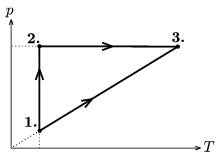Mathematical and Physical Journal
for High Schools
Issued by the MATFUND Foundation
 Already signed up? New to KöMaL?

#Problem P. 3941. (December 2006)

P. 3941. A diatomic ideal gas is taken through the cyclic process shown in the figure. In state 1 the pressure of the gas is 100 kPa and its temperature is 350 K. In state 2 the pressure is 300 kPa. What is the efficiency of the cycle?(5 pont)

Deadline expired on January 11, 2007.

### Statistics:

 100 students sent a solution. 5 points: 52 students. 4 points: 3 students. 3 points: 22 students. 2 points: 12 students. 1 point: 4 students. 0 point: 6 students. Unfair, not evaluated: 1 solutions.

Problems in Physics of KöMaL, December 2006